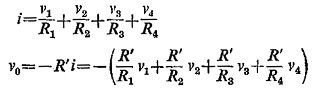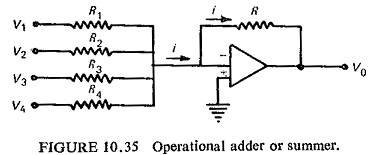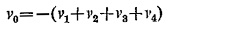## Summation Device:

Summation transformers and sequence networks as already discussed can combine a number of electrical quantities into a single quantity. The operational amplifier is commonly used as a mixer or summer and the Summation Device is shown in Fig. (10.35). The arrangement is used to obtain an output which is a linear combination of a number of input signals.Any desired number of inputs can be used. If a simple summer or adder operation is desired, then R1=R2=R3=R4=R′, such thatMany other methods may, of course, be used to combine signals. The present method has the advantage that it may be extended to a very large number of inputs requiring only one additional resistor for each additional input.

The result depends, in the limiting case of large amplifier gain, only on the resistors involved, and because of the virtual ground, there is a minimum of interaction between input sources.

Scroll to Top